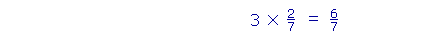S k i l l
i n
A R I T H M E T I C

Lesson 26

# HOW TO MULTIPLY FRACTIONSHOW TO DIVIDE FRACTIONS

 3 × 27 ("3 times 27 ").  What does that mean?

According to the meaning of multiplication,

 3 × 27 = 27 + 27 + 27 .

That is,

 3 × 27 = 2 + 2 + 2       7 = 67 .

We can therefore state the "rule" as follows:

 1. How do we multiply a fraction by a whole number?Multiply the numerator by the whole number. Do not change the denominator. If the fraction becomes improper, extract the whole number.
 Example 1. 2 × 49 = 89 .
 Example 2.   It takes 25 yards of material to make a shirt.

How many yards will it take to make 6 shirts?

 Answer.   6 × 25 = 12 5 = 2 25 yards.

Example 3.  Dividing first.

 18 × 23 = 6 × 2 = 12

"3 goes into (is contained in) 18 six (6) times. 6 times 2 is 12."

The order of multiplying and dividing does not matter. (Property 3 of division.)  If we had multiplied first --

 18 × 23 = 18 × 2    3

-- we would divide by 3 eventually.  But dividing first results in multiplying smaller numbers.

 Example 4. 20 × 11 5 = 4 × 11 = 44

"5 goes into 20 four (4) times."

 Example 5. 3 × 11 3 = 11

In this case, we may simply say that the 3's "cancel."

When the multiplier is a whole number, as 3 in this case, it is never

 necessay to write it as 31 .

 2. How do we multiply a mixed number by a whole number?Multiply the whole number of the mixed number, multiply the fraction.
 2 × 4 = 8. 2 × 13 = 23 .

When multiplying by a whole number, it is never necessary to change to an improper fraction.

This is exactly what we did to multiply dollars and cents (Lesson 9).

4 × \$6.20 = \$24 + \$.80 = \$24 .80

 Example 6. 5 × 3 2 11 = 15 1011 .
 Example 7. 7 × 6 3 5 = 42 21 5 = 42 + 4 15 = 46 15 .

Always, if the fraction becomes improper, extract the whole number.  (Lesson 20.)

 3. How do we multiply a fraction by a fraction?Multiply the numerators and multiply the denominators.

In the next Lesson, we will see what multiplying by a fraction means.

 Example 8. 34 × 7 11 = 2144

When multiplying fractions, do not change to a common denominator.

 g Example 9. 25 × 59 = 29

If any numerator has a divisor in common with any denominator, then, just as in reducing a single fraction, we can divide them by that common divisor.

 Example 10. 89 × 1516

8 and 16 have a common divisor, which is 8 itself.  9 and 15 have a common divisor 3."8 goes into 8 once (1);  8 goes into 16 two (2) times."

"3 goes into 9 three (3) times;  3 goes into 15 five (5) times."

"1 × 5 = 5.   3 × 2 = 6."

Whenever possible it is more skillful to cancel -- reduce -- before multipliying. The fraction will then immediately be in its lowest terms.
If we had multiplied first --

 89 × 1516 = 120144

-- the fraction would be much more difficult to reduce.

 Example 11. 12 × 34 × 57 = 1556

Multiply all the numerators:   1 × 3 × 5 = 15

Multiply all the denominators:   2 × 4 × 7 = 8 × 7 = 56

The time to cancel is before multiplying when the numbers are smaller.  If nothing cancels before , as in this example, then the answer is already in its lowest terms.

 Example 12. 43 × 9 2

2 is a common divisor of 4 and 2.   3 is a common divisor of 3 and 9."2 goes into 4 two (2) times;  2 goes into 2 one (1) time."

"3 goes into 3 one (1) time;  3 goes into 9 three (3) times."

"2 × 3 = 6.   1 × 1 = 1."

 A fraction with denominator 1  ( 61 )  is simply the

numerator. It is not necessary to say, "1 goes into 6 six times."

Here is another example:

 51 = 5.
 Example 13. 4 × 56

We may cancel before multiplying 4 × 5:"2 goes into 4 two (2) times."

"2 goes into 6 three (3) times."

"2 × 5 = 10; over 3."

Since we would find the divisor 2 after multiplying 4 × 5, we can find it even more easily before.  Again, the advantage of reducing first is that we work with smaller numbers.

 4. How do we multiply a mixed number by a mixed number, or a fraction by a mixed number? Change the mixed numbers to improper fractions (Lesson 20).This is the only place in arithmetic where it is necessary to change to an improper fraction.

 Example 15. 2 × 4 13 = 8 23

It is not necessary to change to an improper fraction.  When multiplying by a whole number, multiply the whole number times the whole number, and multiply the whole number times the numerator.

At this point, please "turn" the page and do some Problems.

or

Continue on to the Section 2:  Dividing fractions